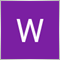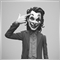# finding price on a trendline ( equation of a straight line)1477

I came across this solution while searching for trendline strategies.

But I am having a slight issue with the code and I do not know why the problem exist. The logic of the code seems correct.
I am interested in this function from the code: ( i have inserted the code)
'int fnGetTrendLineSignal(string strName, int type),'

I have tested this function,
1. I manually drew a trendline on the chart.
2. I entered the trendline name
3. I checked the calculated price( or y value) for the current time (time now). But the calculated price doesn't match the actual price on the trendline for the current time.

I have attached a screenshot of the problem.

i am losing sleep over this.
Thank you very much
```double fnGetTrendLineSignal(string strName)
{
// get the four coordinates
double x1 = ObjectGet( strName, OBJPROP_TIME1);
double y1 = ObjectGet( strName, OBJPROP_PRICE1);
double x2 = ObjectGet( strName, OBJPROP_TIME2);
double y2 = ObjectGet( strName, OBJPROP_PRICE2);

// calculate the slope of the line (m)
double m = (y2-y1)/(x2-x1);

// calcualte the offset (b)
double b=y1 -m*x1;

// get the current x value
double time=TimeCurrent();

// calculate the value (y) of the projected trendline at (x): y = mx + b
double value=m*time+b;

return value;
}```16397

Will Kene:
But I am having a slight issue with the code and I do not know why the problem exist. The logic of the code seems correct.
https://docs.mql4.com/objects/objectgetvaluebyshift20207

Will Kene: . I checked the calculated price( or y value) for the current time (time now). But the calculated price doesn't match the actual price on the trendline for the current time.
```   double x1 = ObjectGet( strName, OBJPROP_TIME1);
double y1 = ObjectGet( strName, OBJPROP_PRICE1);
double x2 = ObjectGet( strName, OBJPROP_TIME2);
double y2 = ObjectGet( strName, OBJPROP_PRICE2);
double m = (y2-y1)/(x2-x1);
double b=y1 -m*x1;
double time=TimeCurrent();
double value=m*time+b;```

Your problem is the assumption of continuous time. You have missing bars (weekend.) Therefor the trendline doesn't have constant slope in time.
"Free-of-Holes" Charts - MQL4 Articles
No candle if open = close ? - MQL4 and MetaTrader 4 - MQL4 programming forum

Compare to

```   double x1 = iBarShift(_Symbol, PERIOD_CURRENT, ObjectGet( strName, OBJPROP_TIME1) );
double y1 = ObjectGet( strName, OBJPROP_PRICE1);
double x2 = iBarShift(_Symbol, PERIOD_CURRENT, ObjectGet( strName, OBJPROP_TIME2) );
double y2 = ObjectGet( strName, OBJPROP_PRICE2);
double m = (y2-y1)/(x2-x1);
double b=y1 -m*x1;
double time=iBarShift(_Symbol, PERIOD_CURRENT, TimeCurrent() );
double value=m*time+b;```1477

whro
Thank you. solves the problem1477

whroeder1:

Your problem is the assumption of continuous time. You have missing bars (weekend.) Therefor the trendline doesn't have constant slope in time.
"Free-of-Holes" Charts - MQL4 Articles
No candle if open = close ? - MQL4 and MetaTrader 4 - MQL4 programming forum

Compare to

Makes sense. Really grateful. Thank you. Now I can sleep. happy pips